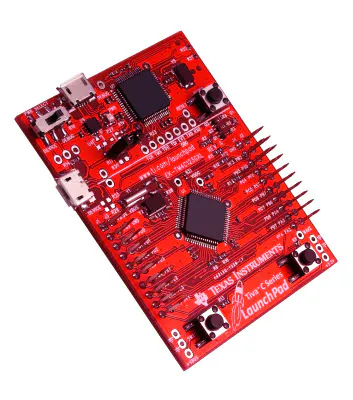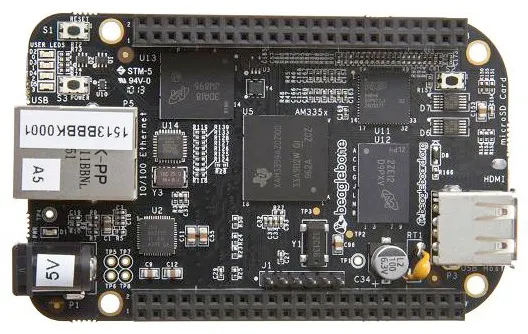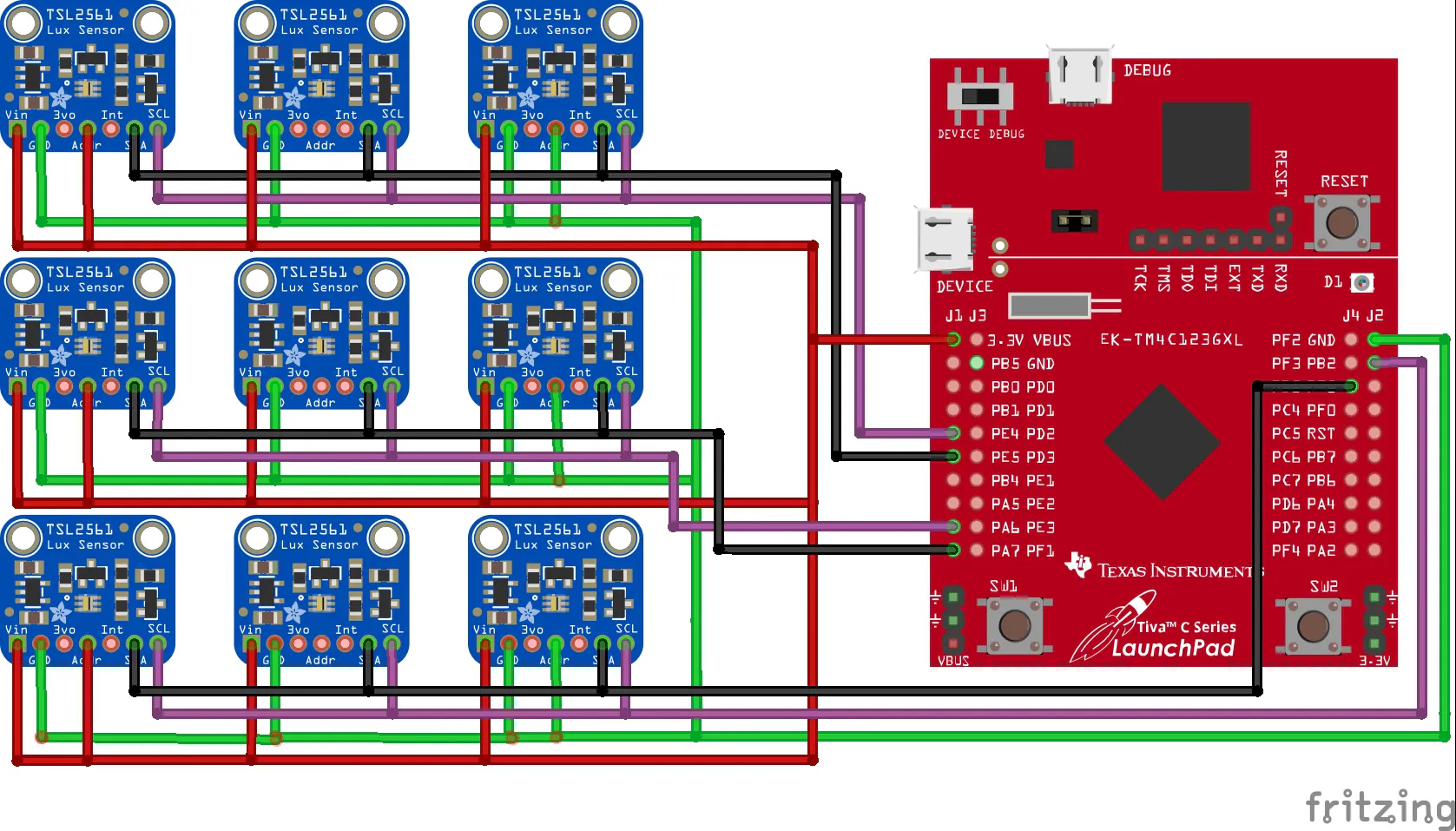# Cloud Motion Vector System for Solar Power Forecasting

Determine a solar power plant's power output using data from the Cloud Motion Vector (CMV) Sensor System and a model of the power plant.

## Things used in this project

### Hardware componentsTexas Instruments EK-TM4C123GXL TM4C Tiva LaunchPad
×1BeagleBoard.org BeagleBone Black
×1
 TSL2561 Light Sensor
×1USB Ethernet Extender, USB to CAT5/6
×1
 4d systems 4DCAPE-70T
×1Ethernet Cable, Cat5e
×1

### Software apps and online servicesTexas Instruments EnergiaMATLAB

### Hand tools and fabrication machines3D Printer (generic)Soldering iron (generic)

## Custom parts and enclosures

### Cube Spacer

Spacers added into the enclosure to ensure stability and rigidity.

### Sensor Base Mount

The sensor is mounted here and placed within the metal enclosure.

### Sample Enclosure

Not really used for the actual project. It's more of like a mockup of the enclosure used.

### Secure Mount for Tiva C Series LaunchPad by kwixson

https://www.thingiverse.com/thing:278816

## Schematics

### CMV Sensor Cluster Schematic## Code

### CMV.sh

SH
```#!/usr/bin/env bash

# This bash script runs 2 python scripts to stream the data from the TM4C123 (TIVA-C)
# then logs the data into an individual CSV at a given hour

## VARIABLES
# end time at 5am UTC = 10pm PST
end_hour=5

## FUNCTIONS
# Start streaming and send process to background
function collectData() {
echo "Starting Data Collection script..."
python /home/ubuntu/pythonCode/getData.py &
}

function sendToServer() {
echo "Starting Thingspeak script..."
python /home/ubuntu/pythonCode/sendToThingspeak.py &
}

function saveData() {
timestamp=\$(date "+%Y-%m-%dT%H%M%S")
cp -a /tmp/temp.csv /home/ubuntu/DATA_COLLECTED/\$timestamp-data.csv
echo "Data collection complete!"

echo "Closing all processes..."
pkill -f getData.py
pkill -f sendToThingspeak.py
echo "Closing complete!"
exit 0
}

## MAIN
echo Starting Cloud Motion Vector sensor...
# Delete file if it exists
if [[ -e /tmp/temp.csv ]]; then
rm /tmp/temp.csv
echo "Renewing temp file complete!"
fi

collectData
sleep 3
sendToServer

while [ 1 ]; do
current_hour=\$(date +%k)
if (( current_hour==end_hour )); then
saveData
else
sleep 60
fi
done
```

### getData.py

Python
```#!/usr/bin/env python
from datetime import datetime

import serial, io, time, shutil

outFile = '/tmp/temp.csv'
ser = serial.Serial(
port='/dev/ttyACM0',
baudrate=115200,
)

sio = io.TextIOWrapper(
io.BufferedRWPair(ser, ser, 1),
encoding='ascii', newline='\r\n'
)

# Give time to initialize port
time.sleep(1)

# "1" here is the start command for the TIVA-C to start collecting data
ser.write('1\n')

with open(outFile,'a') as f: #appends to existing file
while ser.isOpen():
f.write(datetime.utcnow().isoformat() + ',' + datastring)
f.flush() #included to force the system to write to disk

#ser.close()
```

### sendToThingspeak.py

Python
```#!/usr/bin/env python
from collections import deque
import csv, time, schedule
import os
import sys
import urllib            # URL functions
import urllib2           # URL functions

filename="/tmp/temp.csv"
THINGSPEAKKEY = 'INSERTYOURTHINGSPEAKKEYHERE'
THINGSPEAKURL = 'https://api.thingspeak.com/update'

def getLast(csv_filename):
with open(csv_filename, 'r') as f:
try:
except IndexError:  # empty file
lastrow = None
return lastrow

def getLast_v2(csv_filename):
with open(csv_filename, 'r') as f:
lastrow = None
return lastrow

def sendData(url,key,field1,field2,field3,field4,field5,field6,field7,field8,
data1,data2,data3,data4,data5,data6,data7,data8):
"""
Send event to internet site
"""

values = {
'api_key' : key,
'field1' : data1,
'field2' : data2,
'field3' : data3,
'field4' : data4,
'field5' : data5,
'field6' : data6,
'field7' : data7,
'field8' : data8
}

postdata = urllib.urlencode(values)
req = urllib2.Request(url, postdata)

log = time.strftime("%d-%m-%Y,%H:%M:%S") + ","
log = log + data1 + ","
log = log + data2 + ","
log = log + data3 + ","
log = log + data4 + ","
log = log + data5 + ","
log = log + data6 + ","
log = log + data7 + ","
log = log + data8 + ","

try:
# Send data to Thingspeak
response = urllib2.urlopen(req, None, 5)
response.close()
log = log + 'Update ' + html_string

except urllib2.HTTPError, e:
log = log + 'Server could not fulfill the request. Error code: ' + e.code
except urllib2.URLError, e:
log = log + 'Failed to reach server. Reason: ' + e.reason
except:
log = log + 'Unknown error'

print log

if __name__ == "__main__":
while 1:
try:
temp_data=getLast(filename)

data={
"SOUTHWEST":temp_data,
"SOUTH":temp_data,
"SOUTHEAST":temp_data,
"WEST":temp_data,
"ORIGIN":temp_data,
"EAST":temp_data,
"NORTHWEST":temp_data,
"NORTH":temp_data,
"NORTHEAST":temp_data
}

#print data
sendData(THINGSPEAKURL,THINGSPEAKKEY,'field1','field2','field3',
'field4','field5','field6','field7','field8',
data['SOUTHWEST'],data['SOUTH'],data['SOUTHEAST'],data['WEST'],
data['EAST'],data['NORTHWEST'],data['NORTH'],data['NORTHEAST'])
time.sleep(60)
except:
pass
```

### TivaC_TSL2561.ino

Arduino
```#include <Adafruit_Sensor.h>
#include <Wire.h>
#include <flash.h>
#include <hw_types.h>

#define rLED RED_LED // the number of the red LED pin
#define gLED GREEN_LED // the number of the gree LED pin
#define bLED BLUE_LED // the number of the blue LED pin

int count = 0,
arraySize = 0, //buffer array size
commandCode,
buttonState = 0;  // variable for reading the pushbutton status
uint32_t timestamp, //timestamp matrix
data; // data matrix

void configureSensor(void)
{
/* You can also manually set the gain or enable auto-gain support */
Wire.setModule(0);
tsl1.setGain(TSL2561_GAIN_1X);      /* No gain ... use in bright light to avoid sensor saturation */
tsl2.setGain(TSL2561_GAIN_1X);
tsl3.setGain(TSL2561_GAIN_1X);
Wire.setModule(1);
tsl4.setGain(TSL2561_GAIN_1X);      /* No gain ... use in bright light to avoid sensor saturation */
tsl5.setGain(TSL2561_GAIN_1X);
tsl6.setGain(TSL2561_GAIN_1X);
Wire.setModule(2);
tsl7.setGain(TSL2561_GAIN_1X);      /* No gain ... use in bright light to avoid sensor saturation */
tsl8.setGain(TSL2561_GAIN_1X);
tsl9.setGain(TSL2561_GAIN_1X);
/* Changing the integration time gives you better sensor resolution (402ms = 16-bit data) */
Wire.setModule(0);
tsl1.setIntegrationTime(TSL2561_INTEGRATIONTIME_13MS);      /* fast but low resolution */
tsl2.setIntegrationTime(TSL2561_INTEGRATIONTIME_13MS);
tsl3.setIntegrationTime(TSL2561_INTEGRATIONTIME_13MS);
Wire.setModule(1);
tsl4.setIntegrationTime(TSL2561_INTEGRATIONTIME_13MS);      /* fast but low resolution */
tsl5.setIntegrationTime(TSL2561_INTEGRATIONTIME_13MS);
tsl6.setIntegrationTime(TSL2561_INTEGRATIONTIME_13MS);
Wire.setModule(2);
tsl7.setIntegrationTime(TSL2561_INTEGRATIONTIME_13MS);      /* fast but low resolution */
tsl8.setIntegrationTime(TSL2561_INTEGRATIONTIME_13MS);
tsl9.setIntegrationTime(TSL2561_INTEGRATIONTIME_13MS);
}

void setup() {
pinMode(rLED, OUTPUT); // initialize the LED pin as an output
pinMode(gLED, OUTPUT);
pinMode(bLED, OUTPUT);

Serial.begin(115200);
configureSensor();
}

void loop() {
String inString = "";

digitalWrite(gLED, HIGH);   // turn the LED on (HIGH is the voltage level)

// Check and save incoming UART messages
while (Serial.available() > 0) {
if (isDigit(inChar)) {
// convert the incoming byte to a char
// and add it to the string:
inString += (char)inChar;
}
// if you get a newline, print the string,
// then the string's value:
if (inChar == '\n') {
commandCode = inString.toInt();
//Serial.print("MODE:");
//Serial.println(commandCode);
}
}

switch (commandCode) {
case 1: // Collect data and append to CSV format until reset
digitalWrite(gLED, LOW);    // turn the LED off by making the voltage LOW
while (1) {
csvCollect();
}

commandCode = 0; //re-initialize value
break;
case 2: // Collect data and append to TSV format until reset
digitalWrite(gLED, LOW);    // turn the LED off by making the voltage LOW
while (1) {
tsvCollect();
}

commandCode = 0; //re-initialize value
break;
case 3: // Collect data for a set duration as a test
digitalWrite(gLED, LOW);    // turn the LED off by making the voltage LOW

digitalWrite(rLED, HIGH);   // Indicate data collection with red LED
collectData();
digitalWrite(rLED, LOW);    // turn the LED off by making the voltage LOW

commandCode = 0; //re-initialize value
break;
case 4: //
digitalWrite(gLED, LOW);    // turn the LED off by making the voltage LOW

digitalWrite(rLED, HIGH);   // Indicate data collection with red LED
collectData();
digitalWrite(rLED, LOW);    // turn the LED off by making the voltage LOW

digitalWrite(bLED, HIGH);    // Indicate saving with blue LED
flashSave();
digitalWrite(bLED, LOW);    // turn the LED off by making the voltage LOW

commandCode = 0; //re-initialize value
break;
case 5: // check saved data in flash memory
digitalWrite(gLED, LOW);    // turn the LED off by making the voltage LOW
digitalWrite(rLED, HIGH);   // turn the LED on (HIGH is the voltage level
printFlash();
digitalWrite(rLED, LOW);    // turn the LED off by making the voltage LOW

commandCode = 0; //re-initialize value
break;
}
}

void csvCollect() {
uint32_t ts = 0,
lux = 0;
uint16_t full, ir;

int i = 0;

Wire.setModule(0); //get data from CMV bottom row

// SOUTHWEST SENSOR
tsl2.getLuminosity(&full, &ir);
lux = tsl2.calculateLux(full, ir);
Serial.print(lux);
Serial.print(",");

// SOUTH SENSOR
tsl1.getLuminosity(&full, &ir);
lux = tsl1.calculateLux(full, ir);
Serial.print(lux);
Serial.print(",");

//SOUTHEAST SENSOR
tsl3.getLuminosity(&full, &ir);
lux = tsl3.calculateLux(full, ir);
Serial.print(lux);
Serial.print(",");

Wire.setModule(2); //get data from CMV middle row

// WEST SENSOR
tsl8.getLuminosity(&full, &ir);
lux = tsl8.calculateLux(full, ir);
Serial.print(lux);
Serial.print(",");

// ORIGIN SENSOR
tsl7.getLuminosity(&full, &ir);
lux = tsl7.calculateLux(full, ir);
Serial.print(lux);
Serial.print(",");

// EAST SENSOR
tsl9.getLuminosity(&full, &ir);
lux = tsl9.calculateLux(full, ir);
Serial.print(lux);
Serial.print(",");

Wire.setModule(1); //get data from CMV top row

// NORTHWEST SENSOR
tsl5.getLuminosity(&full, &ir);
lux = tsl5.calculateLux(full, ir);
Serial.print(lux);
Serial.print(",");

// NORTH SENSOR
tsl4.getLuminosity(&full, &ir);
lux = tsl4.calculateLux(full, ir);
Serial.print(lux);
Serial.print(",");

// NORTHEAST SENSOR
tsl6.getLuminosity(&full, &ir);
lux = tsl6.calculateLux(full, ir);
Serial.println(lux);
}

void tsvCollect() {
uint32_t ts = 0,
lux = 0;
uint16_t full, ir;

int i = 0;

Wire.setModule(0);

ts = millis();
tsl1.getLuminosity(&full, &ir);
lux = tsl1.calculateLux(full, ir);
Serial.print(ts);
Serial.print(" ");
Serial.print(lux);
Serial.print(" ");

ts = millis();
tsl2.getLuminosity(&full, &ir);
lux = tsl2.calculateLux(full, ir);
Serial.print(ts);
Serial.print(" ");
Serial.print(lux);
Serial.print(" ");

ts = millis();
tsl3.getLuminosity(&full, &ir);
lux = tsl3.calculateLux(full, ir);
Serial.print(ts);
Serial.print(" ");
Serial.print(lux);
Serial.print(" ");

Wire.setModule(1);

ts = millis();
tsl4.getLuminosity(&full, &ir);
lux = tsl4.calculateLux(full, ir);
Serial.print(ts);
Serial.print(" ");
Serial.print(lux);
Serial.print(" ");

ts = millis();
tsl5.getLuminosity(&full, &ir);
lux = tsl5.calculateLux(full, ir);
Serial.print(ts);
Serial.print(" ");
Serial.print(lux);
Serial.print(" ");

ts = millis();
tsl6.getLuminosity(&full, &ir);
lux = tsl6.calculateLux(full, ir);
Serial.print(ts);
Serial.print(" ");
Serial.print(lux);
Serial.print(" ");

Wire.setModule(2);

ts = millis();
tsl7.getLuminosity(&full, &ir);
lux = tsl7.calculateLux(full, ir);
Serial.print(ts);
Serial.print(" ");
Serial.print(lux);
Serial.print(" ");

ts = millis();
tsl8.getLuminosity(&full, &ir);
lux = tsl8.calculateLux(full, ir);
Serial.print(ts);
Serial.print(" ");
Serial.print(lux);
Serial.print(" ");

ts = millis();
tsl9.getLuminosity(&full, &ir);
lux = tsl9.calculateLux(full, ir);
Serial.print(ts);
Serial.print(" ");
Serial.println(lux);
}

void collectData() {
uint32_t startTime = 0, //start time of data collection in seconds
currentTime = 0, //current time in milliseconds
elapsedTime = 0, //time since collection started
endTime = 20000; //data collection end time in milliseconds
uint16_t full, ir;
int i, j;

// Initialize counter
count = 0;

// Initialize timestamp and data arrays to zero
for (i = 0; i < 200; i++) {
for (j = 0; j < 9; j++) {
timestamp[i][j] = 0;
data[i][j] = 0;
}
}

startTime = millis(); //get start time

while (elapsedTime < endTime) {
//Serial.print(currentTime);
currentTime = millis();
elapsedTime = currentTime - startTime;
Wire.setModule(0);

// Get sensor data
timestamp[count] = millis();
tsl1.getLuminosity(&full, &ir);
data[count] = tsl1.calculateLux(full, ir);
Serial.print(timestamp[count]);
Serial.print(" ");
Serial.print(data[count]);
Serial.print(" ");

timestamp[count] = millis();
tsl2.getLuminosity(&full, &ir);
data[count] = tsl2.calculateLux(full, ir);
Serial.print(timestamp[count]);
Serial.print(" ");
Serial.print(data[count]);
Serial.print(" ");

timestamp[count] = millis();
tsl3.getLuminosity(&full, &ir);
data[count] = tsl3.calculateLux(full, ir);
Serial.print(timestamp[count]);
Serial.print(" ");
Serial.print(data[count]);
Serial.print(" ");

Wire.setModule(1);

timestamp[count] = millis();
tsl4.getLuminosity(&full, &ir);
data[count] = tsl4.calculateLux(full, ir);
Serial.print(timestamp[count]);
Serial.print(" ");
Serial.print(data[count]);
Serial.print(" ");

timestamp[count] = millis();
tsl5.getLuminosity(&full, &ir);
data[count] = tsl5.calculateLux(full, ir);
Serial.print(timestamp[count]);
Serial.print(" ");
Serial.print(data[count]);
Serial.print(" ");

timestamp[count] = millis();
tsl6.getLuminosity(&full, &ir);
data[count] = tsl6.calculateLux(full, ir);
Serial.print(timestamp[count]);
Serial.print(" ");
Serial.print(data[count]);
Serial.print(" ");

Wire.setModule(2);

timestamp[count] = millis();
tsl7.getLuminosity(&full, &ir);
data[count] = tsl7.calculateLux(full, ir);
Serial.print(timestamp[count]);
Serial.print(" ");
Serial.print(data[count]);
Serial.print(" ");

timestamp[count] = millis();
tsl8.getLuminosity(&full, &ir);
data[count] = tsl8.calculateLux(full, ir);
Serial.print(timestamp[count]);
Serial.print(" ");
Serial.print(data[count]);
Serial.print(" ");

timestamp[count] = millis();
tsl9.getLuminosity(&full, &ir);
data[count] = tsl9.calculateLux(full, ir);
Serial.print(timestamp[count]);
Serial.print(" ");
Serial.println(data[count]);

count++;
}
}

void flashSave() {
/* Save data and timestamp into memory */
flashData = 0,
pui32data; //buffer;
int i = 0,
j = 0,
k = 0;

// Initialize data buffer
for (i = 0; i < 3000; i++) {
pui32data[i] = 0;
}

for (i = 0; i < count; i++) {
for (j = 0; j < 9; j++) {
pui32data[k] = timestamp[i][j]; //copy timestamp into buffer
k++; //increment counter

pui32data[k] = data[i][j]; //copy data into buffer
k++; //increment counter
}
}
arraySize = k;

/* Populate data buffer to be sent to memory */
FlashErase(addr); // erase flash memory block
FlashErase(0x3400); // erase flash memory block
FlashErase(0x3600); // erase flash memory block
FlashErase(0x3800); // erase flash memory block
FlashErase(0x3C00); // erase flash memory block
FlashErase(0x4000); // erase flash memory block
FlashErase(0x4400); // erase flash memory block
FlashErase(0x4600); // erase flash memory block
FlashErase(0x4800); // erase flash memory block
FlashErase(0x4C00); // erase flash memory block
FlashErase(0x5000); // erase flash memory block
FlashErase(0x5400); // erase flash memory block
FlashProgram(pui32data, addr, sizeof(pui32data)); //write data into flash memory

for (i = 0; i < arraySize; i++) {
flashData = HWREG(addr + (i * 4));
Serial.println(flashData);
}

Serial.println(" ");
Serial.println(arraySize);
Serial.println(sizeof(pui32data));
}

void printFlash() {
tFlashProtection fx;

Serial.println("\nPrinting FLASH permission map.");

for (i = 0; i < 255; ++i) {

Serial.print("@");
Serial.print("\t");
switch (fx) {
Serial.println("Flash can be read and written!");
break;
break;
case (FlashExecuteOnly):
Serial.println("Flash can only be executed!");
default:
Serial.println("Unknown tFlashProtection value!");
}
}
}

void printHexBytes(byte * adr, uint32_t n) {
uint32_t i, j, k;
const int deflen = 16;
char c;
char str[deflen];

//Serial.println("");
for (i = 0; i < n;) {
//Serial.print("\t");
Serial.print("\t");
for (j = i, k = 0; j < (i + deflen); ++j, ++k) {
if (c < 16)
Serial.print("  ");
else
Serial.print(" ");
if (c >= 32 && c <= 126)
str[k] = c;
else
str[k] = '.';
}
i += deflen;
Serial.print("\t");
for (j = 0; j < deflen; ++j) {
Serial.print(str[j]);
}
Serial.print("\n");
}
}
```

## Credits

### HyperChiicken

11 projects • 27 followers
I cobble things together :]